# Initialize Clustering Model (GMM) VI

LabVIEW 2018 Analytics and Machine Learning Toolkit Help

Edition Date: July 2018

Part Number: 377059B-01

»View Product InfoDownload Help (Windows Only)

Owning Palette: Clustering VIs

Requires: Analytics and Machine Learning Toolkit

Initializes the hyperparameters of the Gaussian mixture model (GMM) algorithm. You can either directly set the hyperparameters or specify multiple values for each hyperparameter. If you specify multiple values for each hyperparameter, the Train Clustering Model VI uses grid search to find the optimal set of hyperparameters.

Use the pull-down menu to select an instance of this VI.

 Select an instance Set ParametersSearch Parameters

## Set Parameters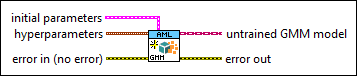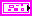initial parameters specifies the initial parameters to train the GMM model.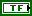auto initialization specifies whether this VI automatically calculates the values in initial parameters using K-Means algorithm. The default is TRUE, which specifies that this VI automatically sets the values in initial parameters using K-Means algorithm.

If you do not specify values for the arrays in initial parameters and auto initialization is FALSE, this VI randomly calculates the values in initial parameters.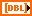initial mean values specifies the initial mean values of all Gaussian distributions in the GMM model.initial covariance values specifies the initial covariance values of all Gaussian distributions in the GMM model.initial covariance matrix specifies the initial covariance matrix of each Gaussian distribution in the GMM model.initial weights specifies the initial weights of all Gaussian distributions in the GMM model.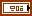hyperparameters specifies the hyperparameters to train the GMM model.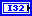number of clusters specifies the number of Gaussian distributions in the GMM model. The default is 3.regulator specifies the value that this VI adds to the covariance matrix to prevent the covariance matrix from converging to 0. The default is 0.000001.tolerance specifies the tolerance for the stopping criteria. The default is 0.0001. The model fitting stops if the change of maximum likelihood between two successive optimization iterations is lower than tolerance.max iteration specifies the maximum number of optimization iterations for the stopping criteria. The default is 100. The model fitting stops if the number of optimization iterations reaches max iteration.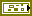error in describes error conditions that occur before this node runs. This input provides standard error in functionality.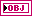untrained GMM model returns the initialized GMM model for training.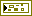error out contains error information. This output provides standard error out functionality.

## Search Parametersinitial parameters specifies the initial parameters to train the GMM model.auto initialization specifies whether this VI automatically calculates the values in initial parameters using K-Means algorithm. The default is TRUE, which specifies that this VI automatically sets the values in initial parameters using K-Means algorithm.

If you do not specify values for the arrays in initial parameters and auto initialization is FALSE, this VI randomly calculates the values in initial parameters.initial mean values specifies the initial mean values of all Gaussian distributions in the GMM model.initial covariance values specifies the initial covariance values of all Gaussian distributions in the GMM model.initial covariance matrix specifies the initial covariance matrix of each Gaussian distribution in the GMM model.initial weights specifies the initial weights of all Gaussian distributions in the GMM model.hyperparameter grids specifies multiple values for each hyperparameter of the GMM model.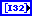number of clusters specifies the number of Gaussian distributions in the trained GMM model.regulator specifies the value that this VI adds to covariance to prevent the covariance matrix from converging to 0.tolerance specifies the tolerance for the stopping criteria.max iteration specifies the maximum number of optimization iterations for the stopping criteria. The model fitting stops if the number of optimization iterations reaches max iteration.attempts specifies the number of times that the Train Clustering Model VI trains the model with different hyperparameter combinations.hyperparameter optimization specifies the method of optimization to determine the optimal hyperparameter settings.hyperparameter search method specifies the method to search for the optimal set of hyperparameters.

 0 Exhaustive search (default)—Tests all possible hyperparameter combinations in the training process. This method is reliable but time-consuming. 1 Random search—Tests a random subset of the hyperparameter combinations in the training process. This method is faster.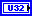number of searchings specifies the number of hyperparameter combinations that this VI selects to train the model. The default is 1. This input is valid only if hyperparameter search method is Random search.evaluation metric specifies the criterion to evaluate the trained clustering model.

 0 Davies Bouldin Index—Evaluates the clustering model using the Davies Bouldin Index metric. The lower the value of metric, the better the compactness and separation of the clustering model. 1 Dunn Index—Evaluates the clustering model using the Dunn Index metric. The higher the value of metric, the better the compactness and separation of the clustering model. 2 Jaccard Index—Evaluates the clustering model using the Jaccard Index metric. The lower the value of metric, the better the separation of the clustering model. 3 Rand Index—Evaluates the clustering model using the Rand Index metric. The lower the value of metric, the better the separation of the clustering model. 4 AIC (default)—Evaluates the GMM model using the Akaike Information Criterion (AIC) metric. The lower the value of metric, the better the GMM model is. 5 BIC—Evaluates the GMM model using the Bayesian Information Criterion (BIC) metric. The lower the value of metric, the better the GMM model is.error in describes error conditions that occur before this node runs. This input provides standard error in functionality.untrained GMM model returns the initialized GMM model for training.error out contains error information. This output provides standard error out functionality.

## Initialize Clustering Model (GMM) Details

The following equation defines the GMM model:where K is the number of Gaussian mixture components. Each Gaussian mixture component represents a Gaussian distribution. μi and Σi are the mean value and the covariance value of the ith Gaussian mixture component αi is the weight of the ith Gaussian mixture component

## Examples

Refer to the following VIs for examples of using the Initialize Clustering Model (GMM) VI:

• Clustering (Set Parameters, Training) VI: labview\examples\AML\Clustering
• Clustering (Search Parameters, Training) VI: labview\examples\AML\Clustering